## The equation of motion of a missile are x = 3t, y = –4t, z = t, where the time ‘t’ is given in seconds, and the distance is measured in kilometres.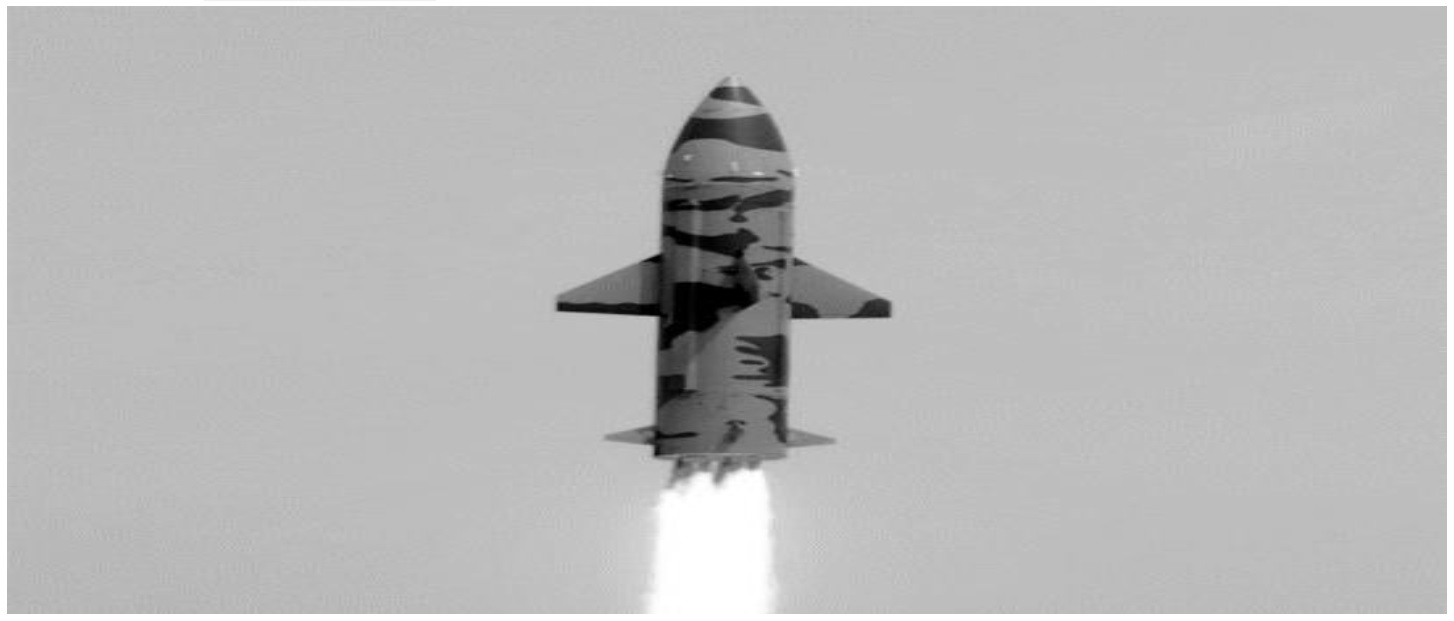(adsbygoogle = window.adsbygoogle || []).push({}); Based on the above answer the following: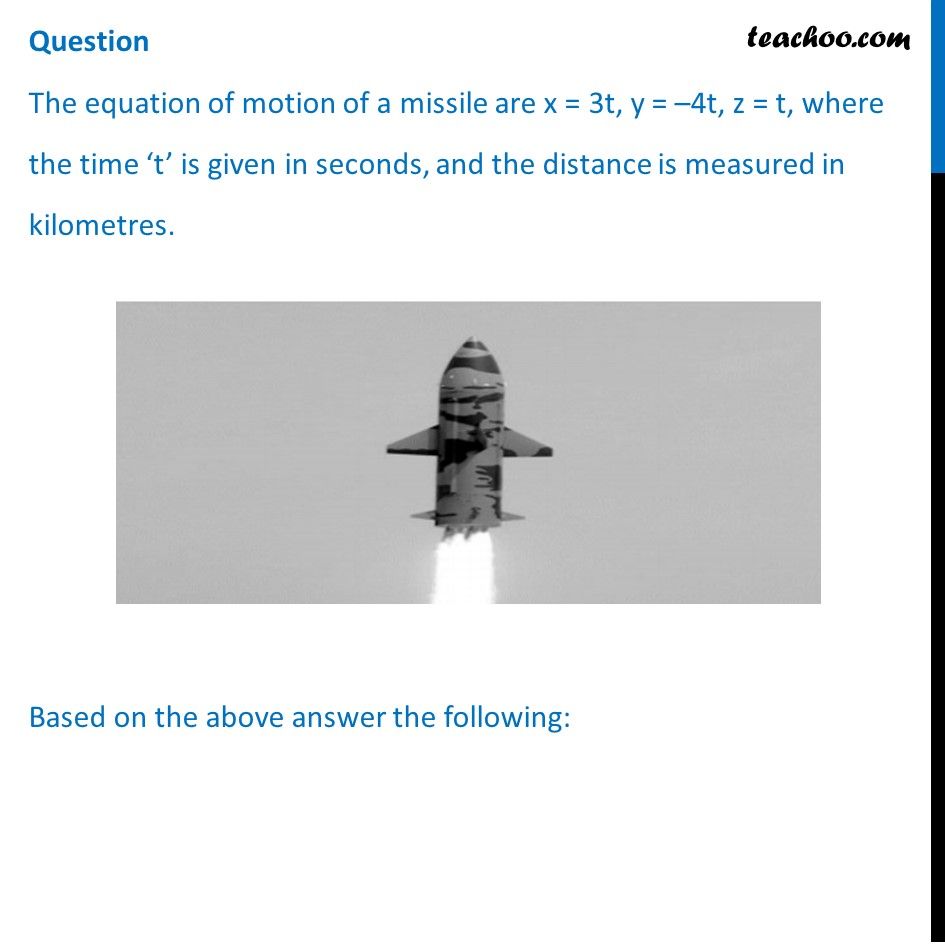## (d) Ellipse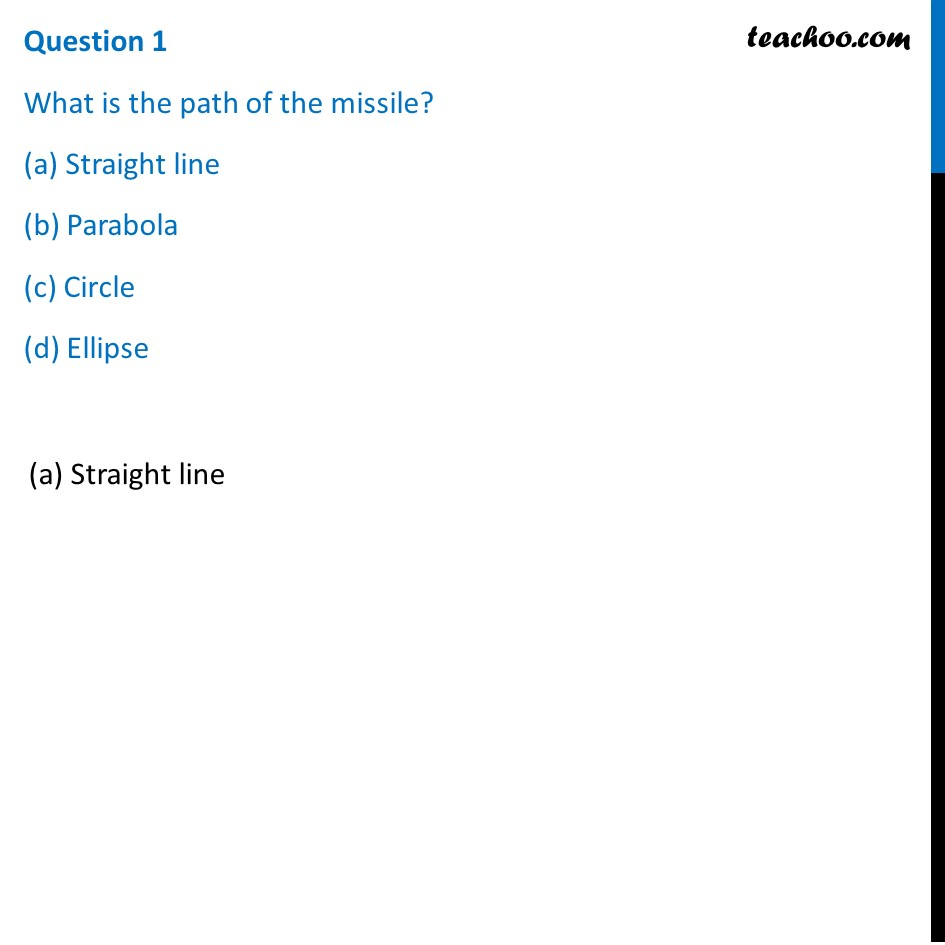## (d) (–6, –8, 2)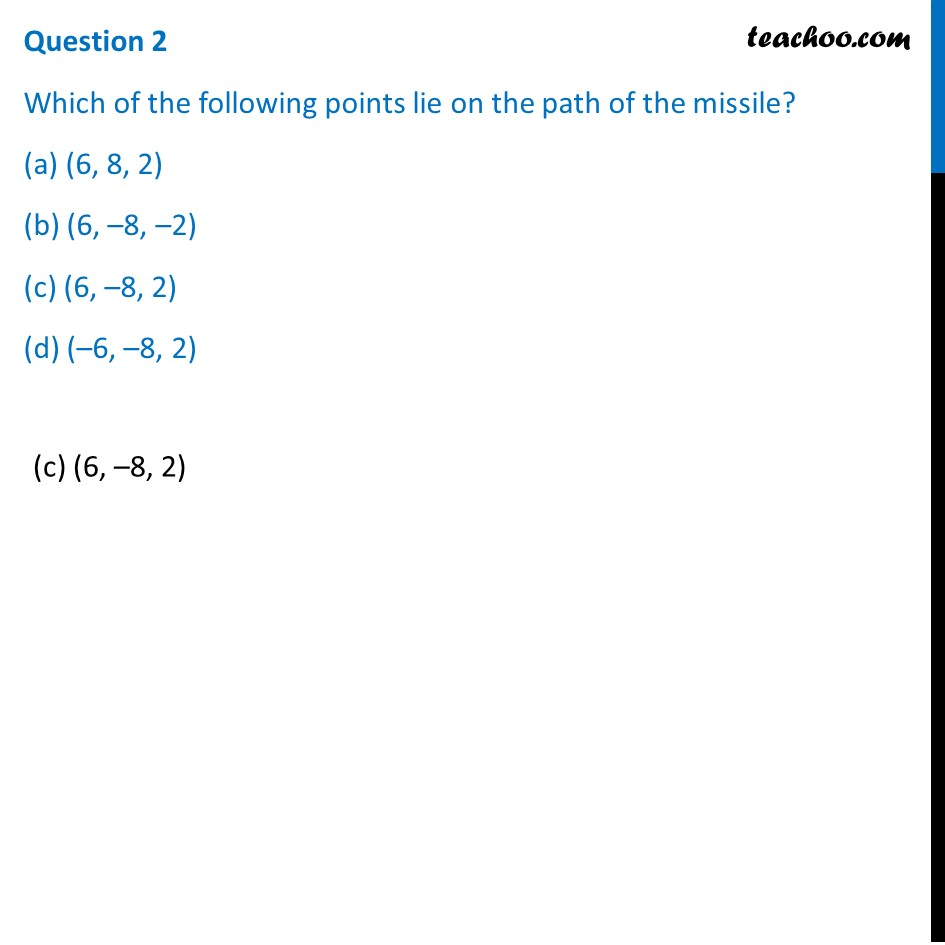## (d) √750 kms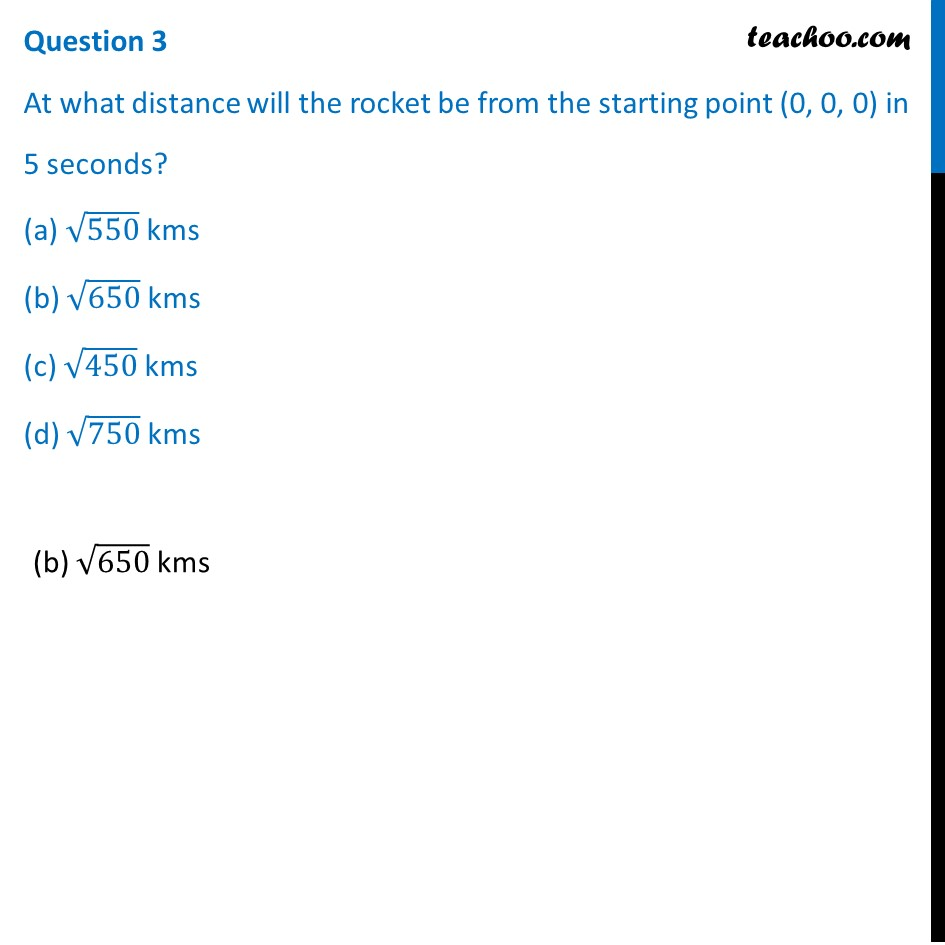## (d) 10 km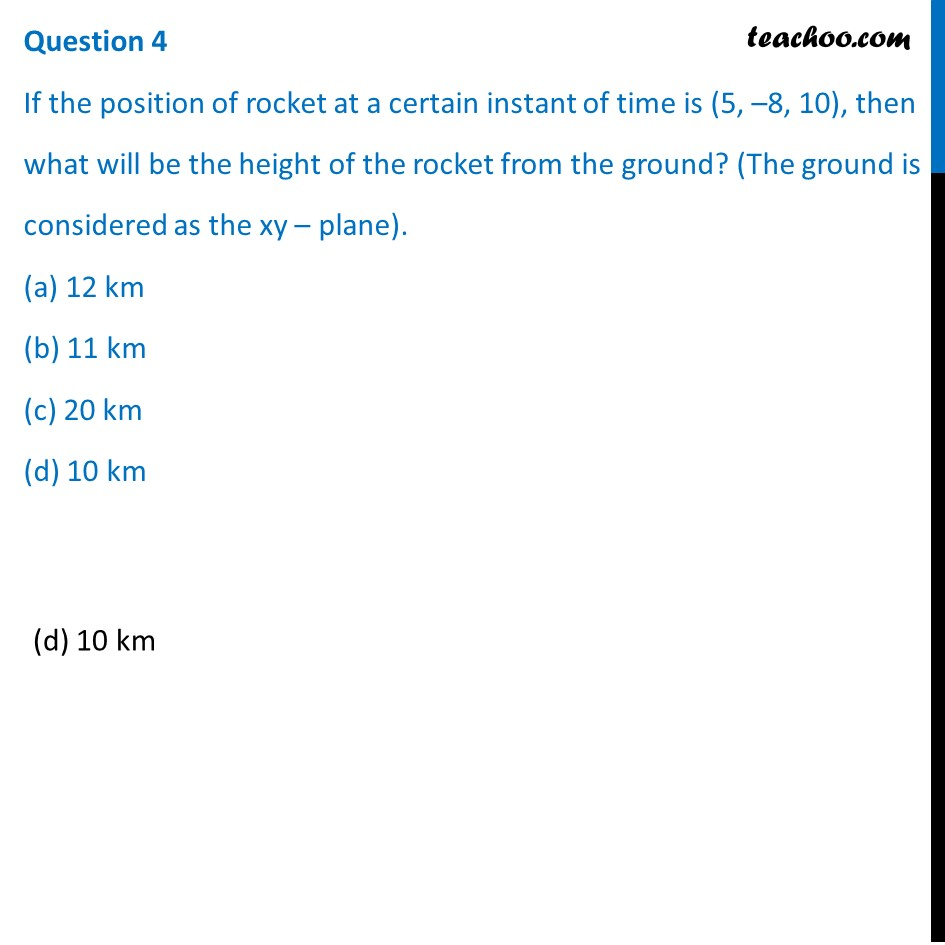## (d) ((-9)/7,(-1)/7,10/7)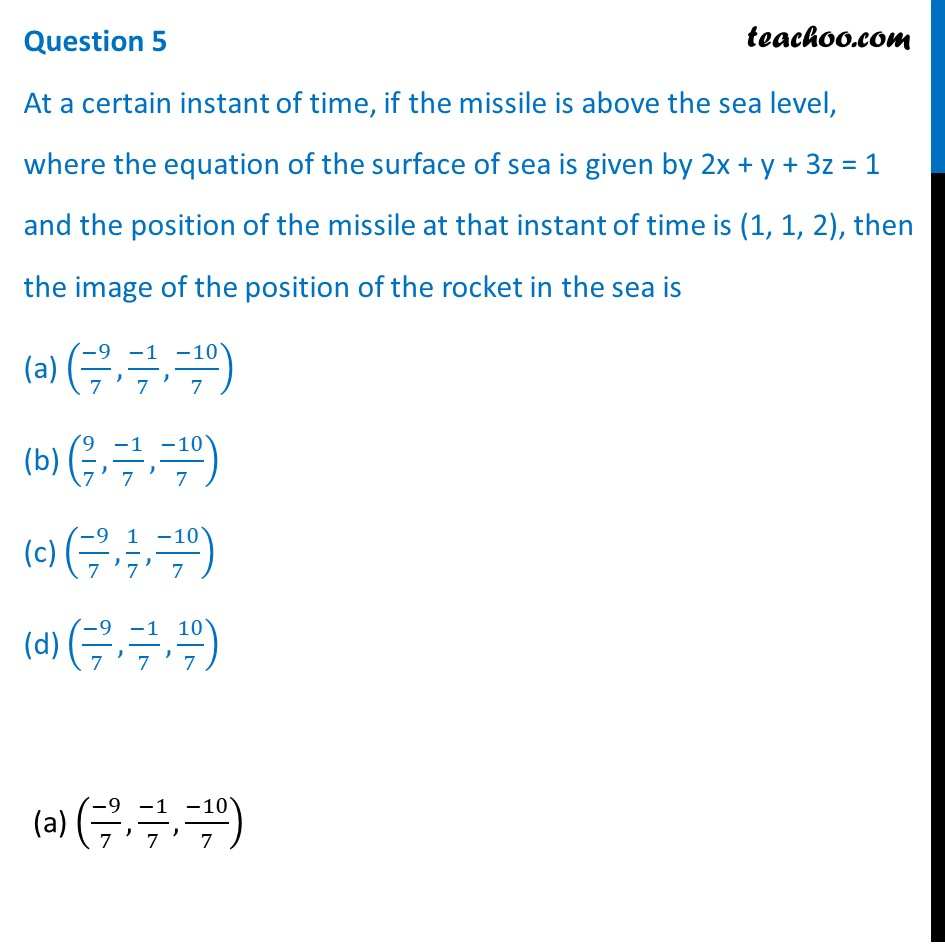1. Chapter 11 Class 12 Three Dimensional Geometry (Term 2)
2. Serial order wise
3. Case Based Questions (MCQ)

Transcript

Question The equation of motion of a missile are x = 3t, y = –4t, z = t, where the time ‘t’ is given in seconds, and the distance is measured in kilometres. Based on the above answer the following:Question 1 What is the path of the missile? (a) Straight line (b) Parabola (c) Circle (d) Ellipse(a) Straight line Question 2 Which of the following points lie on the path of the missile? (a) (6, 8, 2) (b) (6, –8, –2) (c) (6, –8, 2) (d) (–6, –8, 2) (c) (6, –8, 2) Question 3 At what distance will the rocket be from the starting point (0, 0, 0) in 5 seconds? (a) √550 kms (b) √650 kms (c) √450 kms (d) √750 kms (b) √650 kms Question 4 If the position of rocket at a certain instant of time is (5, –8, 10), then what will be the height of the rocket from the ground? (The ground is considered as the xy – plane). (a) 12 km (b) 11 km (c) 20 km (d) 10 km (d) 10 km Question 5 At a certain instant of time, if the missile is above the sea level, where the equation of the surface of sea is given by 2x + y + 3z = 1 and the position of the missile at that instant of time is (1, 1, 2), then the image of the position of the rocket in the sea is (a) ((−9)/7,(−1)/7,(−10)/7) (b) (9/7,(−1)/7,(−10)/7) (c) ((−9)/7,1/7,(−10)/7) (d) ((−9)/7,(−1)/7,10/7)(a) ((−9)/7,(−1)/7,(−10)/7)

Case Based Questions (MCQ)